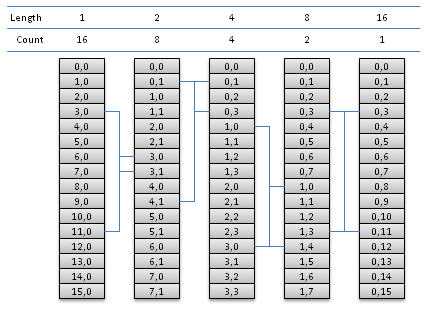# OpenCL Fast Fourier Transform

Eric Bainville - May 2010, updated March 2011

As a starting point, we will implement a simple out of place radix-2 where, at each step, all sequences are stored in contiguous entries of the arrays. Input and output buffers are in global memory: each step requires the execution of an instance of the kernel.Radix-2 DFT, N=16. For each iteration, we show the contents (a,k)Length of the current array, a is the start index of the transformed sequence, and k the value index in the DFT. Count is the number of sub-sequences, and Length is their length. For each iteration, an example of radix-2 butterfly is displayed (blue lines). Butterfly inputs are always at distance 8=N/2 in the array, and outputs are at distance Length.

If we run one thread for each butterfly taking indices i and i+N/2 as inputs, all memory read operations will always be coalesced (1 single memory access per group of 16 threads executed at the same time) as soon as N/2 is large enough. Memory write operations will be coalesced for all iterations but the first few.

The kernel operates on buffer x and stores the output in y. The next iteration is then called with (y,x), etc.

Let's first define some basic functions and macros:

```// Return A*B
real2_t mul(real2_t a,real2_t b)
{
#else
#endif
}

// Return A * exp(K*ALPHA*i)
real2_t twiddle(real2_t a,int k,real_t alpha)
{
real_t cs,sn;
sn = sincos((real_t)k*alpha,&cs);
return mul(a,(real2_t)(cs,sn));
}

// In-place DFT-2, output is (a,b). Arguments must be variables.
#define DFT2(a,b) { real2_t tmp = a - b; a += b; b = tmp; }
```

```// Compute T x DFT-2.
// T is the number of threads.
// N = 2*T is the size of input vectors.
// X[N], Y[N]
// P is the length of input sub-sequences: 1,2,4,...,T.
// Each DFT-2 has input (X[I],X[I+T]), I=0..T-1,
// and output Y[J],Y|J+P], J = I with one 0 bit inserted at postion P. */
__kernel void fftRadix2Kernel(__global const real2_t * x,__global real2_t * y,int p)
{
int t = get_global_size(0); // thread count
int i = get_global_id(0);   // thread index
int k = i&(p-1);            // index in input sequence, in 0..P-1
int j = ((i-k)<<1) + k;     // output index
real_t alpha = -FFT_PI*(real_t)k/(real_t)p;

x += i;
real2_t u0 = x;
real2_t u1 = twiddle(x[t],1,alpha);

// In-place DFT-2
DFT2(u0,u1);

// Write output
y += j;
y = u0;
y[p] = u1;
}
```

This kernel must be called for p=1, then p=2, etc. until p=N/2. The number of threads at each call is N/2, and the work group size WG doesn't matter since all threads are independent. We need to insert a barrier between two kernel executions, or use OpenCL events to make sure the output array is entirely updated before being used as input in the next call.

In the next page, we will merge two radix-2 iterations into one radix-4 iteration: Higher radix kernels.